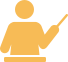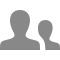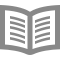## Probability Theory, Statistics and Exploratory Data Analysis

Price:Free

###### Date: On request

Type: e-learning

Category: Data Collection & Analytics

Language: English

City: Online

### Training Description:

Exploration of Data Science requires certain background in probability and statistics. This course introduces you to the necessary sections of probability theory and statistics, guiding you from the very basics all way up to the level required for jump starting your ascent in Data Science.

The core concept of the course is random variable ? i.e. variable whose values are determined by random experiment. Random variables are used as a model for data generation processes we want to study. Properties of the data are deeply linked to the corresponding properties of random variables, such as expected value, variance and correlations. Dependencies between random variables are crucial factor that allows us to predict unknown quantities based on known values, which forms the basis of supervised machine learning. We begin with the notion of independent events and conditional probability, then introduce two main classes of random variables: discrete and continuous and study their properties. Finally, we learn different types of data and their connection with random variables. While introducing you to the theory, we'll pay special attention to practical aspects for working with probabilities, sampling, data analysis, and data visualization in Python. This course requires basic knowledge in Discrete mathematics (combinatorics) and calculus (derivatives, integrals).

### Speakers:

Instructor rating4.8/5 (64 Ratings)### Ilya V. Schurov

Associate Professor31,413 Learners5 Courses

Data analysis

## Participation

To participate Enroll Now